# How to Calculate years between dates in Excel

In this article, we will learn about how to get years between two dates using YEARFRAC function in Excel.

The YEARFRAC function returns the number of years elapsed between the two dates. It returns a decimal value.
Syntax:

=YEARFRAC(start_date, end_date)

start_date : Start date
end_date : End date

Example:
Let’s understand this function using it in an example.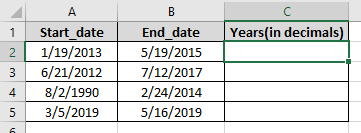Here we have the start_date and end_date in two columns Use the formula to get the years between two dates:

=YEARFRAC(A2,B2)

A2 : start_date
B2 : end_date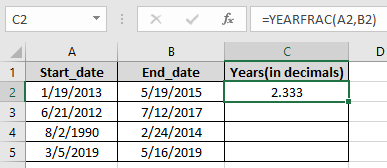As you can see, it returns the number of Years between the two dates

Copy the formula in other cells using Ctrl + D shortcut key.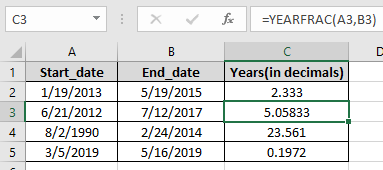The formula returns the number of years in decimal values.

Now use the ROUND function on the result values to get the years in Integers.
Use the formula:

=ROUND(C2,0)

C2 : number to be round off
0 : round off upto num_digit.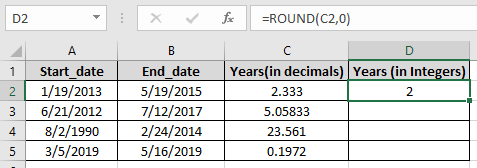As you can see, it returns the number of Years in Integers between the two dates

Copy the formula in other cells using Ctrl + D shortcut key.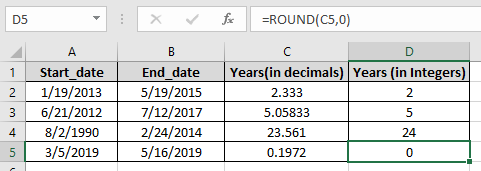You can use the INT function, ROUNDUP function or TRUNC function on the YEARFRAC function to get the round off results.
Use the formula to get the number in Integers:

=TRUNC(YEARFRAC(A2,B2), 0)

YEARFRAC(A2,B2) : returns the number of years in decimal
0 : upto decimal places.

As you can see we have the truncated results for the given values

Hope you understood how to use TRUNC function in Excel. Explore more articles on Excel Text editing function here. Please feel free to state your query or feedback for the above article.

Related Articles:

How to Use the YEAR Function in Excel

How to Add Years to a Date in Excel

How to Calculate days, months and years in Excel

How to Calculate days, years and months from certain date in Excel

Popular Articles:

How to use the VLOOKUP Function in Excel

How to use the COUNTIF function in Excel

How to use the SUMIF Function in Excel

Terms and Conditions of use

The applications/code on this site are distributed as is and without warranties or liability. In no event shall the owner of the copyrights, or the authors of the applications/code be liable for any loss of profit, any problems or any damage resulting from the use or evaluation of the applications/code.